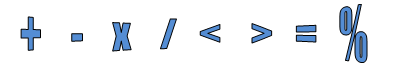# BT Easy Math Reference Guide A guide for math homework help and in class

“The gold in this guide is found in the three pages devoted to word problems. Many children seem to get hung up on word problems. If your child has trouble in this area, I think the ideas here would be a big help. Strategies include looking for clue words, drawing a diagram, and working backwards. Lots of examples of various types of word problems are illustrated step-by-step.

Kathy G, The Old Schoolhouse Magazine

“So often when we are doing a lesson we have to read the hard math book first in order to get it, now I don’t have to sit and read the ‘at times obscure chapter’ to see what to do. This shows me in an easy to understand format. This is so easy for me to use with my students and for my students and for the parents of my students. It is teacher friendly, parent-friendly and student friendly. And it’s cool that I can keep it in a binder so it is always handy. Thank you so much for creating this.”

Gail S, parent and teacher, CA

The ultimate cheat sheet for any math assignment… It even helps those with dyslexia, dyscalulia & ADHD Starting with addition and subtraction, this 16-page guide shows step by step how to solve all elementary math calculations right up to fractions and decimals. Also covered are all those “other” math topics, such as word problems, bar and circle graph, rounding and estimating, place value, geometry, measurements, money, and averaging.

\$17

## Math Homework Help is Here

• 16 pages packed with all the math you child or student needs to know 1st – 8th!
• GREAT as a refresher for all parents
• Step-by-step how to solve math homework problems.

### The BT Easy Math Reference Guide Includes:

• Subtraction
• Multiplication
• Division
• Fractions
• Decimals
• Percents
• Word Problem Strategies
• Math Vocabulary and much, much, more!
•  And, it fits in your binder!

## Give Your Child the Tools to be Successful with Math and Math HomeworkMost children have gaps in their understanding of calculations with: fractions, decimals, percents, or word problems. Without these basics, once they’re in Algebra and beyond, they tend to feel hopelessly lost. I wanted to be sure my children and students would be able to understand the math concepts and be successful with their math assignments. I also wanted them to have something to refer to if I wasn’t there to offer guidance to them. So, I decided to create the same type of reference guide for their math homework assignments that I did to help them with their writing assignments (the Writer’s Easy Reference Guide). This time I would address the roadblocks to math success. The math homework reference guide would be filled with all of the basics of elementary school math. The BT Easy Math Reference Guide would bridge the gap between the theoretical and the practical. It can also help you become your child’s Math Guru and be so easy to use that your child will even be able to use it on their own.

## Math Homework Help

Did you know that in math assignments that contain word problems there are clue words in every word problem? And if you understand what the clue words are telling you what to do, you will be able to solve any word problem. That is why I explain what the clue words are telling you and give you examples of just about every type of word problem there is. That way your child can just match their math problem up with the example and see how to do it.

### Imagine the day when your child can accurately:

• Calculate fractions, decimals, percents
• Solve word problems
• Calculate area, perimeter, and ratio with ease.
• Recognize and do calculations with geometric shapes, lines, and angles.

I must admit, I got a bit carried away when I was developing the BT Easy Math Reference Guide, so it is 16 pages packed with all the math you child needs to know for 1st through 8th grade math! It’s GREAT as a refresher for all parents trying to remember how to help their child solve their homework problem.